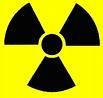Science Quiz / 'M' Units of Measurement

Random Science or Math Quiz

Can you name the units of measurement that begin with 'm'?

byCryptus  Plays Quiz not verified by Sporcle

Score
0/31
Timer
09:00
ClueUnit
A measure of relative velocity, used to express the speed of an aircraft relative to the speed of sound.
A unit used either in astronomy to express the brightness of stars, or in earth science to express the intensity of earthquakes.
A unit of volume for wine, equal to 1.5 litres.
A unit of length used in athletics, equal to 26 miles, 385 yards.
A CGS unit of magnetic flux, equal to 10-8 weber.
A unit used in music to represent a series of beats; also called a bar.
A unit of information, commonly equivalent to 1,000,000 bytes, but sometimes also equivalent to 1,048,576 bytes.
A unit of frequency equal to 1 million per second; commonly seen in stating radio wave frequencies.
An empirical scale used for rating the effects of an earthquake, rather than its intensity.
A unit used to measure the fineness of woven products such as fishing nets or window screens, equal to the number of strands per inch.
The SI unit of length.
The SI unit of velocity.
A unit of volume for wine, equal to 6 litres.
A unit of distance equal to 10-6 metres.
A unit of distance equal to 1760 yards.
A unit of fuel efficiency.
An imperial unit of velocity.
A unit of time equal to 1000 years.
A unit of atmospheric pressure, equivalent to a hectopascal.
A unit of mass equal to 1000 micrograms, commonly used in medicinal dosages.
A unit of volume, equivalent to 1 cubic centimetre.
A unit of distance, equal to 0.1 centimetres.
A unit of pressure, equal to the pressure exerted at the Earth's surface by a column of mercury 1 millimeter high; often used in medicine to record blood pressure.
A unit of work or energy, representing the energy delivered at a rate of one milliwatt for a period of one hour.
A unit of relative time in music equal to 1/2 a whole note.
A unit of time or angle, equal to 1/60.
A scale used to express the hardness of a mineral.
The SI base unit of amount of substance.
A medieval unit of time equal to 1/40 of an hour; now used to simply mean a short amount of time.
A unit of time, roughly equal to 1/12 of a year.
A unit of genetic separation used in genetics and biotechnology.

From the Vault See Another

Sporcle Minesweeper XI
Who else had Minesweeper installed on one of those old off-white clunker computers?

Extras

Created Jan 18, 2016
Curator Picked Curator Pick (Physics): Jan 20, 2016